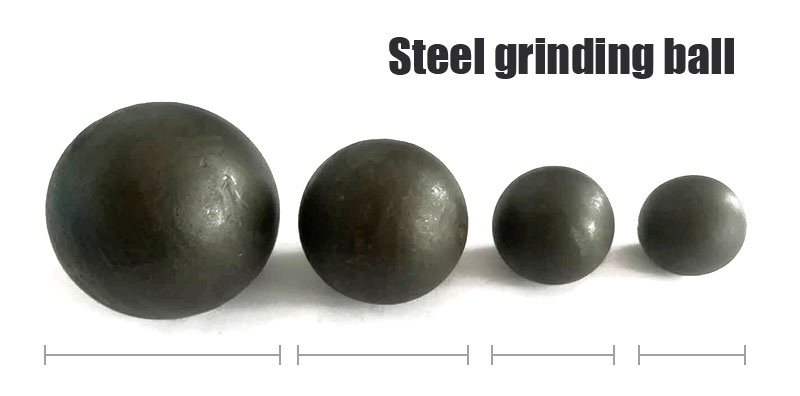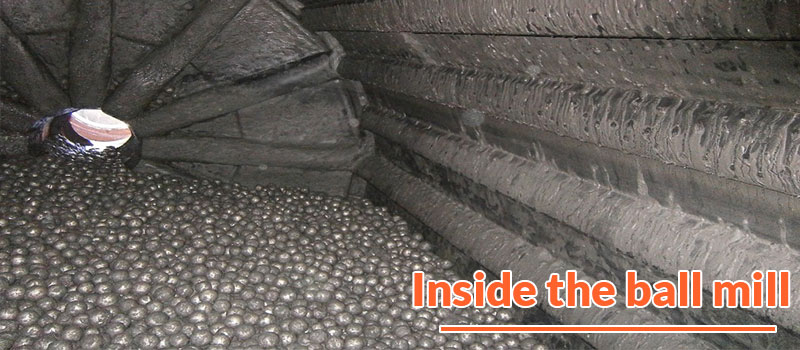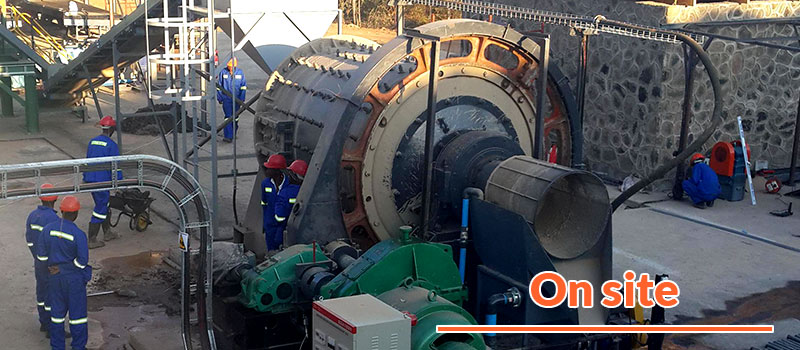﻿ Method of Steel Grinding Ball Proportioning and Example | Fote Machinery
1. Home >
2. Blog >
3. Blog details

# Method of Steel Grinding Ball Proportioning and Example

Author : Jordan Last Updated :

A ball mill is equipped with a variety of balls of different sizes to process mixed materials with different sizes. In order to obtain a good grinding effect, the mass ratio of various balls should be compatible with the particle size of materials.

## What is steel grinding ball proportioning?Steel grinding ball proportioning in a ball mill has two implications. One is to determine which types of balls to install. The other is to determine the proportion of balls of various diameters, and what their actual mass is.

New processing plants can learn from similar beneficiation plants on the actual ball addition ratio, then make an appropriate adjustment in production.

## Method of Steel grinding ball proportioningThe method of ball distribution for a ball mill can be summarized as follows:

1. Take all the ore samples from a ball mill (usually take new ore and returned sand samples, then match the samples in proportion) for sieving analysis. Divide them into several particle sizes, such as 18-12, 12-10, 10-8, 8-6, etc. Then weigh each particle size, and calculate the mass percentage (yield) of each grain size, such as γ18-12，γ12-10, etc.;

2. Use the upper limit of each particle size (such as 18, 12, 10, 8, etc.) or the average value of the upper and lower limits (such as 15, 11, 9, 7, etc.) to refer to Table 18 or use the formula to determine the ball diameter that needs to be added for each particle size, such as γ18-12，γ12-10, etc.;

3. The mass ratio of various balls should be compatible with the particle size of materials. Determine the percentage of various sizes of balls in the total mass of balls added, corresponding to γ' D18= γ18-12、γ' D12= γ12-10、γ' D10= γ10-8、γ' D8= γ8-6, etc.;

4. Adjust the calculation results appropriately. Because too small balls are not matched in actual ball allocation, we only select several kinds of balls. Therefore, the calculated ball with a small diameter can be allocated to the larger ball in proportion. The mass percentage of each particle size of balls can be re-determined, such as γD18，γD12, γD10, etc.;

5. Calculate the actual mass of each particle size of balls.

a. Calculate the total mass G of balls according to the formula:

G = π / 4 × D2 × L × ϕ × δ

In this formula, G means the total mass of the ball(t). D is the inner diameter of ball mill cylinder(m). L is the effective length of the cylinder of the ball mill(m). ϕ is the medium filling rate(%). And δ is the bulk density of the ball(t /m3).

b. Then calculate the mass of each ball.

GD18=G • γD18，GD12=G • γD12，GD10=G • γD10，GD8=G • γD8 ,etc.

## Example of steel grinding ball proportioning

A beneficiation plant in Singapore loads balls into a ϕ 1500mm×3000mm wet grid type ball mill, determines the filling rate ϕ = 50%, and selects cast steel grinding balls δ = 4.5 t/m3. The ball mill processes the medium-hard ore.1. See Table 1 for the sieving analysis results of all the ore samples from a ball mill.

Table 1

 Grain size (mm) Yield (%) Grain size (mm) Yield (%) 18-12 γ18-12 =20 6-4 γ6-4 =5 12-10 γ12-10 =40 4-2 γ4-2 =4 10-8 γ10-8 =15 2-1 γ2-1 =4 8-6 γ8-6 =8 1-0 γ1-0 =4

2. Calculate the ball diameter according to the upper limit of each particle size and round up. See Table 2.

Table 2

 Grain size (mm) Ball diameter (mm) Grain size (mm) Ball diameter (mm) 18-12 D18 =120 6-4 D6 =70 12-10 D12 =100 4-2 D4 =60 10-8 D10 =90 2-1 D2 =50 8-6 D8 =80 1-0 D1-0 =40

3. The mass percentages of various balls are shown in Table 21.

Table 21

 Ball diameter (mm) Quality ratio (%) Ball diameter (mm) Quality ratio (%) 120 20 70 5 100 40 60 4 90 15 50 4 80 8 40 4

If it is determined that only four balls of 120, 100, 80 and 60 are added, the ratio of 90, 70, 50 and 40 balls can be appropriately adjusted to other balls. The adjusted results of this example are shown in Table 3.

Table 3

 Ball diameter (mm) Quality ratio (%) Ball diameter (mm) Quality ratio (%) 120 30 80 13 100 45 60 12

4. Calculate the total mass G of the added balls:

G = π / 4 × D2 × L × ϕ × δ = π / 4 × 1.52 × 3 × 0.5 × 4.5 = 11.928 (t)

5. Calculate the mass of each ball that should be added separately.

G120 = G • γ120 =11.928 x 30% = 3.58 (t)

G100 = G • γ100 =11.928 x 45% = 5.37 (t)

G80 = G • γ80 =11.928 x 13% = 1.55 (t)

G60 = G • γ60 =11.928 x 12% = 1.43 (t)

6. According to the calculation result, weigh the ball.Author : Jordan Jordan is the writer of the blog with a broad knowledge of this industry. Most importantly, he hopes to help you in your projects sincerely.

As a leading mining machinery manufacturer and exporter in China, we are always here to provide you with high quality products and better services. Welcome to contact us through one of the following ways or visit our company and factories.

Your email will only for contact and not be shared with any third parties.

• Product Name
• Name
• Email*
• Phone or Whatsapp
• Please enter the material to be processed, expected capacity,feeding size (mm), final product size (mm), or other requirements.

Fote is a manufacturer of high-end mining machinery in Asia.

contact@sinoftm.com
FTM Machinery-Green Intelligent Mining Machine Manufacturing and Exporting Base

Based on the high quality and complete after-sales service, our products have been exported to more than 120 countries and regions. Fote Machinery has been the choice of more than 200,000 customers.

•Provide 7 * 24 hours after-sales
consultation service

•The overseas service team can
arrive at the site within a week

•Promise one-year guarantee
period and free maintenance

INTERESTED IN OUR EQUIPMENT?